# Resources tagged with: Place value

Filter by: Content type:
Age range:
Challenge level:

### There are 85 results

Broad Topics > Numbers and the Number System > Place value##### Age 5 to 11 Challenge Level:

Try out this number trick. What happens with different starting numbers? What do you notice?### Oddly

##### Age 7 to 11 Challenge Level:

Find the sum of all three-digit numbers each of whose digits is odd.### Six Is the Sum

##### Age 7 to 11 Challenge Level:

What do the digits in the number fifteen add up to? How many other numbers have digits with the same total but no zeros?### Napier's Bones

##### Age 7 to 11 Challenge Level:

The Scot, John Napier, invented these strips about 400 years ago to help calculate multiplication and division. Can you work out how to use Napier's bones to find the answer to these multiplications?### Being Resourceful - Primary Number

##### Age 5 to 11 Challenge Level:

Number problems at primary level that require careful consideration.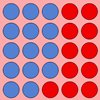### Place Value as a Building Block for Developing Fluency in the Calculation Process

##### Age 5 to 11

This article for primary teachers encourages exploration of two fundamental ideas, exchange and 'unitising', which will help children become more fluent when calculating.### Spell by Numbers

##### Age 7 to 11 Challenge Level:

Can you substitute numbers for the letters in these sums?### ABC

##### Age 7 to 11 Challenge Level:

In the multiplication calculation, some of the digits have been replaced by letters and others by asterisks. Can you reconstruct the original multiplication?### Trebling

##### Age 7 to 11 Challenge Level:

Can you replace the letters with numbers? Is there only one solution in each case?### Being Resilient - Primary Number

##### Age 5 to 11 Challenge Level:

Number problems at primary level that may require resilience.### Alien Counting

##### Age 7 to 11 Challenge Level:

Investigate the different ways these aliens count in this challenge. You could start by thinking about how each of them would write our number 7.### Being Collaborative - Primary Number

##### Age 5 to 11 Challenge Level:

Number problems at primary level to work on with others.### All the Digits

##### Age 7 to 11 Challenge Level:

This multiplication uses each of the digits 0 - 9 once and once only. Using the information given, can you replace the stars in the calculation with figures?### Multiply Multiples 2

##### Age 7 to 11 Challenge Level:

Can you work out some different ways to balance this equation?### Multiply Multiples 1

##### Age 7 to 11 Challenge Level:

Can you complete this calculation by filling in the missing numbers? In how many different ways can you do it?### Multiply Multiples 3

##### Age 7 to 11 Challenge Level:

Have a go at balancing this equation. Can you find different ways of doing it?### Number Detective

##### Age 5 to 11 Challenge Level:

Follow the clues to find the mystery number.### Round the Three Dice

##### Age 7 to 11 Challenge Level:

What happens when you round these three-digit numbers to the nearest 100?### Journeys on the Gattegno Tens Chart

##### Age 5 to 11

Alf describes how the Gattegno chart helped a class of 7-9 year olds gain an awareness of place value and of the inverse relationship between multiplication and division.### One Million to Seven

##### Age 7 to 11 Challenge Level:

Start by putting one million (1 000 000) into the display of your calculator. Can you reduce this to 7 using just the 7 key and add, subtract, multiply, divide and equals as many times as you like?### Diagonal Sums

##### Age 7 to 11 Challenge Level:

In this 100 square, look at the green square which contains the numbers 2, 3, 12 and 13. What is the sum of the numbers that are diagonally opposite each other? What do you notice?### Which Scripts?

##### Age 7 to 11 Challenge Level:

There are six numbers written in five different scripts. Can you sort out which is which?### Subtraction Surprise

##### Age 7 to 14 Challenge Level:

Try out some calculations. Are you surprised by the results?### Which Is Quicker?

##### Age 7 to 11 Challenge Level:

Which is quicker, counting up to 30 in ones or counting up to 300 in tens? Why?### The Thousands Game

##### Age 7 to 11 Challenge Level:

Each child in Class 3 took four numbers out of the bag. Who had made the highest even number?### Song Book

##### Age 7 to 11 Challenge Level:

A school song book contains 700 songs. The numbers of the songs are displayed by combining special small single-digit cards. What is the minimum number of small cards that is needed?##### Age 5 to 11 Challenge Level:

Who said that adding couldn't be fun?### More Upper Primary Activities on Number Sense and Place Value

##### Age 7 to 11

More upper primary number sense and place value tasks.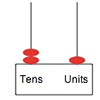### Position

##### Age 5 to 11

One of the key ideas associated with place value is that the position of a digit affects its value. These activities support children in understanding this idea.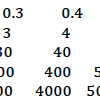### Number Sense and Place Value

##### Age 5 to 11

This feature aims to support you in developing children's early number sense and understanding of place value.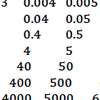### How Can I Support the Development of Early Number Sense and Place Value?

##### Age 3 to 11

This article for primary teachers expands on the key ideas which underpin early number sense and place value, and suggests activities to support learners as they get to grips with these ideas.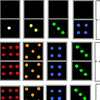### Ordering

##### Age 5 to 11

This set of activities focuses on ordering, an important aspect of place value.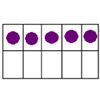### Place Value: the Ten-ness of Ten

##### Age 3 to 11

This article develops the idea of 'ten-ness' as an important element of place value.### Coded Hundred Square

##### Age 7 to 11 Challenge Level:

This 100 square jigsaw is written in code. It starts with 1 and ends with 100. Can you build it up?### Activities on the Gattegno Chart

##### Age 5 to 11

In this article, Alf outlines six activities using the Gattegno chart, which help to develop understanding of place value, multiplication and division.### Becky's Number Plumber

##### Age 7 to 11 Challenge Level:

Becky created a number plumber which multiplies by 5 and subtracts 4. What do you notice about the numbers that it produces? Can you explain your findings?### Football Sum

##### Age 11 to 14 Challenge Level:

Find the values of the nine letters in the sum: FOOT + BALL = GAME### Round the Dice Decimals 2

##### Age 7 to 11 Challenge Level:

What happens when you round these numbers to the nearest whole number?### Round the Dice Decimals 1

##### Age 7 to 11 Challenge Level:

Use two dice to generate two numbers with one decimal place. What happens when you round these numbers to the nearest whole number?### Cayley

##### Age 11 to 14 Challenge Level:

The letters in the following addition sum represent the digits 1 ... 9. If A=3 and D=2, what number is represented by "CAYLEY"?### What Do You Need?

##### Age 7 to 11 Challenge Level:

Four of these clues are needed to find the chosen number on this grid and four are true but do nothing to help in finding the number. Can you sort out the clues and find the number?### Digit Sum

##### Age 11 to 14 Challenge Level:

What is the sum of all the digits in all the integers from one to one million?### Tis Unique

##### Age 11 to 14 Challenge Level:

This addition sum uses all ten digits 0, 1, 2...9 exactly once. Find the sum and show that the one you give is the only possibility.### Arrange the Digits

##### Age 11 to 14 Challenge Level:

Can you arrange the digits 1,2,3,4,5,6,7,8,9 into three 3-digit numbers such that their total is close to 1500?### Two and Two

##### Age 11 to 14 Challenge Level:

How many solutions can you find to this sum? Each of the different letters stands for a different number.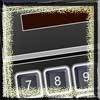### Calculator Bingo

##### Age 7 to 11 Challenge Level:

A game to be played against the computer, or in groups. Pick a 7-digit number. A random digit is generated. What must you subract to remove the digit from your number? the first to zero wins.### Chocolate Maths

##### Age 11 to 14 Challenge Level:

Pick the number of times a week that you eat chocolate. This number must be more than one but less than ten. Multiply this number by 2. Add 5 (for Sunday). Multiply by 50... Can you explain why it. . . .##### Age 11 to 14 Challenge Level:

Powers of numbers behave in surprising ways. Take a look at some of these and try to explain why they are true.### Being Curious - Primary Number

##### Age 5 to 11 Challenge Level:

Number problems for inquiring primary learners.### Reach 100

##### Age 7 to 14 Challenge Level:

Choose four different digits from 1-9 and put one in each box so that the resulting four two-digit numbers add to a total of 100.